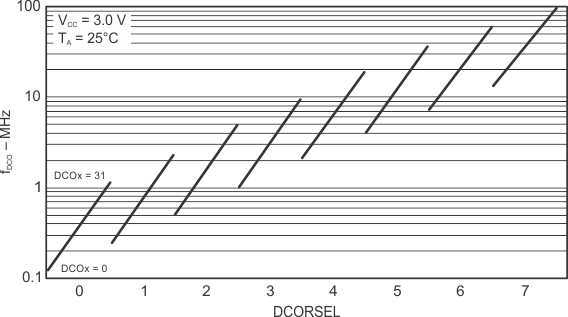SLAS590P March   2009  – September 2020

PRODUCTION DATA

• ZXH|80
• RGC|64
• RGC|64

## 8.19 DCO Frequency

over recommended ranges of supply voltage and operating free-air temperature (unless otherwise noted)
PARAMETERTEST CONDITIONSMINTYPMAXUNIT
fDCO(0,0)DCO frequency (0, 0)(1)DCORSELx = 0, DCOx = 0, MODx = 00.070.20MHz
fDCO(0,31)DCO frequency (0, 31)(1)DCORSELx = 0, DCOx = 31, MODx = 00.701.70MHz
fDCO(1,0)DCO frequency (1, 0)(1)DCORSELx = 1, DCOx = 0, MODx = 00.150.36MHz
fDCO(1,31)DCO frequency (1, 31)(1)DCORSELx = 1, DCOx = 31, MODx = 01.473.45MHz
fDCO(2,0)DCO frequency (2, 0)(1)DCORSELx = 2, DCOx = 0, MODx = 00.320.75MHz
fDCO(2,31)DCO frequency (2, 31)(1)DCORSELx = 2, DCOx = 31, MODx = 03.177.38MHz
fDCO(3,0)DCO frequency (3, 0)(1)DCORSELx = 3, DCOx = 0, MODx = 00.641.51MHz
fDCO(3,31)DCO frequency (3, 31)(1)DCORSELx = 3, DCOx = 31, MODx = 06.0714.0MHz
fDCO(4,0)DCO frequency (4, 0)(1)DCORSELx = 4, DCOx = 0, MODx = 01.33.2MHz
fDCO(4,31)DCO frequency (4, 31)(1)DCORSELx = 4, DCOx = 31, MODx = 012.328.2MHz
fDCO(5,0)DCO frequency (5, 0)(1)DCORSELx = 5, DCOx = 0, MODx = 02.56.0MHz
fDCO(5,31)DCO frequency (5, 31)(1)DCORSELx = 5, DCOx = 31, MODx = 023.754.1MHz
fDCO(6,0)DCO frequency (6, 0)(1)DCORSELx = 6, DCOx = 0, MODx = 04.610.7MHz
fDCO(6,31)DCO frequency (6, 31)(1)DCORSELx = 6, DCOx = 31, MODx = 039.088.0MHz
fDCO(7,0)DCO frequency (7, 0)(1)DCORSELx = 7, DCOx = 0, MODx = 08.519.6MHz
fDCO(7,31)DCO frequency (7, 31)(1)DCORSELx = 7, DCOx = 31, MODx = 060135MHz
SDCORSELFrequency step between range DCORSEL and DCORSEL + 1SRSEL = fDCO(DCORSEL+1,DCO)/fDCO(DCORSEL,DCO)1.22.3ratio
SDCOFrequency step between tap DCO and DCO + 1SDCO = fDCO(DCORSEL,DCO+1)/fDCO(DCORSEL,DCO)1.021.12ratio
Duty cycleMeasured at SMCLK40%50%60%
dfDCO/dTDCO frequency temperature drift(2)fDCO = 1 MHz0.1%/°C
dfDCO/dVCCDCO frequency voltage drift(3)fDCO = 1 MHz1.9%/V
When selecting the proper DCO frequency range (DCORSELx), the target DCO frequency, fDCO, should be set to reside within the range of fDCO(n, 0),MAX ≤ fDCO ≤ fDCO(n, 31),MIN, where fDCO(n, 0),MAX represents the maximum frequency specified for the DCO frequency, range n, tap 0 (DCOx = 0) and fDCO(n,31),MIN represents the minimum frequency specified for the DCO frequency, range n, tap 31 (DCOx = 31). This ensures that the target DCO frequency resides within the range selected. It should also be noted that if the actual fDCO frequency for the selected range causes the FLL or the application to select tap 0 or 31, the DCO fault flag is set to report that the selected range is at its minimum or maximum tap setting.
Calculated using the box method: (MAX(–40°C to 85°C) – MIN(–40°C to 85°C)) / MIN(–40°C to 85°C) / (85°C – (–40°C))
Calculated using the box method: (MAX(1.8 V to 3.6 V) – MIN(1.8 V to 3.6 V)) / MIN(1.8 V to 3.6 V) / (3.6 V – 1.8 V)Figure 8-10 Typical DCO Frequency